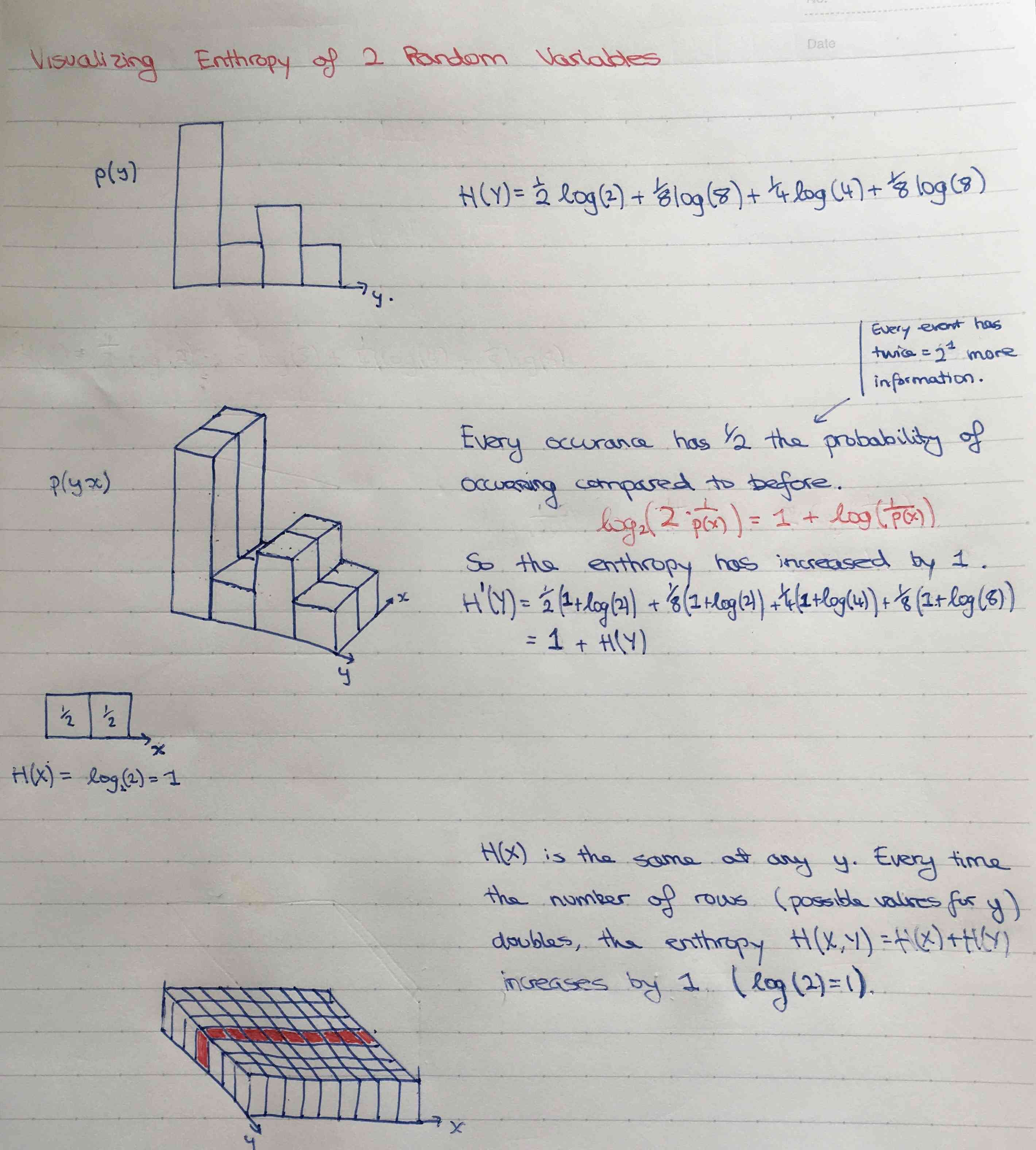Show Question
Joint entropy of two random variables

For two ensembles, $$X = (x, A_x, P_x)$$ and $$Y = (y, A_y, P_y)$$, where there may be dependency between $$P_x$$ and $$P_y$$, the joint entropy of $$X$$, $$Y$$ is:

$H(X, Y) = \sum_{x \in A_x} \sum_{y \in A_y} P(x, y)log \frac{1}{P(x, y)}$

Entropy is additive for independent random variables.

#### Proof

\begin{align*} H(X, Y) &= \sum_{x \in A_x} \sum_{y \in A_y} P(x)P(y)log\frac{1}{P(x)P(y)} \\ &= \sum_{x \in A_x} \sum_{y \in A_y} P(x)P(y)log\frac{1}{P(x)} +\sum_{x \in A_x} \sum_{y \in A_y} P(x)P(y)log\frac{1}{P(y)} \\ &=\sum_{x \in A_x}P(x)log\frac{1}{P(x)} + \sum_{y \in A_y} P(y)log\frac{1}{P(y)} \text{ (the first sum's terms are independent of y, and the second's independent of x)}\\ &= H(X) + H(Y)\end{align*}